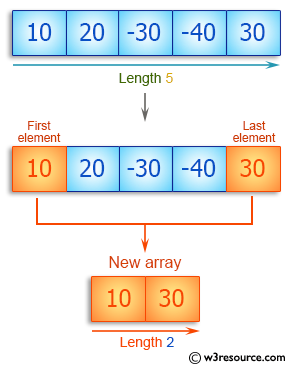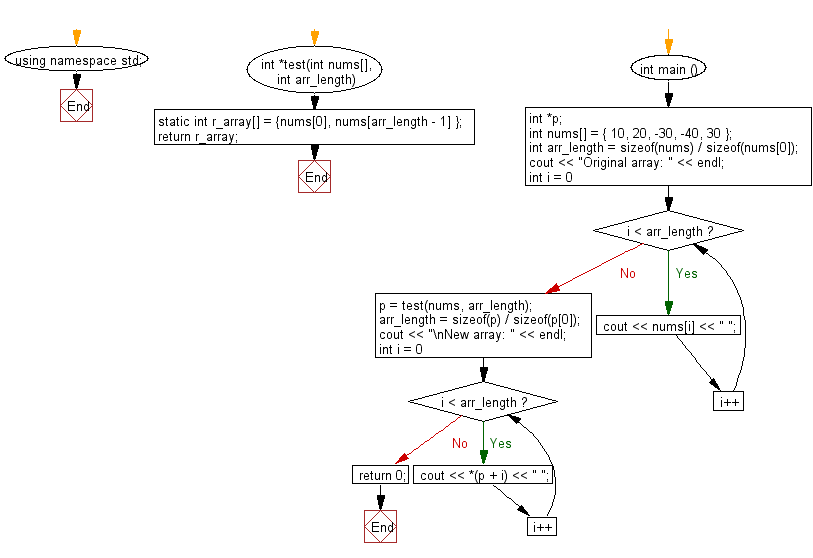﻿ C++ : Create new from the first, last elements of an array# C++ Exercises: Create a new array taking the first and last elements of a given array of integers and length 1 or more

## C++ Basic Algorithm: Exercise-88 with Solution

Write a C++ program to create a new array taking the first and last elements of a given array of integers and length 1 or more.

Sample Solution:

C++ Code :

``````#include <iostream>
using namespace std;

int *test(int nums[], int arr_length) {
static int  r_array[]
= {nums, nums[arr_length - 1] };
return r_array;
}

int main () {
// a pointer to an int.
int *p;
int nums[] = { 10, 20, -30, -40, 30 };

int arr_length = sizeof(nums) / sizeof(nums);
cout << "Original array: " << endl;
for ( int i = 0; i < arr_length; i++ ) {
cout << nums[i] << " ";
}

p = test(nums, arr_length);
arr_length = sizeof(p) / sizeof(p);
cout << "\nNew array: " << endl;
for ( int i = 0; i < arr_length; i++ ) {
cout << *(p + i) << " ";
}

return 0;
}
``````

Sample Output:

```Original array:
10 20 -30 -40 30
New array:
10 30
```

Pictorial Presentation:Flowchart:C++ Code Editor: